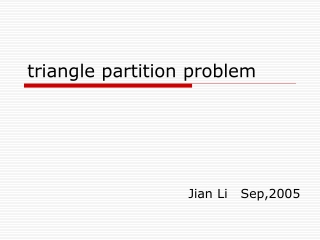DownloadDownload Presentationtriangle partition problem

# triangle partition problem

Download Presentation## triangle partition problem

- - - - - - - - - - - - - - - - - - - - - - - - - - - E N D - - - - - - - - - - - - - - - - - - - - - - - - - - -
##### Presentation Transcript

1. triangle partition problem Jian Li Sep,2005

2. Proposed by Redstar in Algorithm board in Fudan BBS. • Motivated by some network design strategy.

3. Problem description • 3n point in a metric space. • A triangle partition is to divide this 3n points into n point disjoint triangle. • GOAL: Obtain a triangle partition T to minimize maxt2 T{e2 tlen(e)}

4. NP-hard proof • Reduction from 3-partition problem • 3-partition problem: • Given 3n integers. The question is whether we can partition them into n class, where each class contain exactly 3 integers, such that the sums of integers in each class are all the same.

5. Reduction: • Create 3n nodes. Each node vi represent a integer ai. • Define the distance len(vi,vj)=ai+aj • It is easy to verify it is a metric len(vi,vj)+len(vj,vk)· len(vi,vk) • We claim the {a1………a3n} admits a 3-partition if and only if maxt2 T{e2 tlen(e)}·i=13nai/n

6. A failed attempt • If we have a polynomial algorithm to do the following thing, we can optimally solve the problem.(of course we can’t) • Suppose oracle A solve the following problem:For a graph G(V,E) with 3n vertice, can we partition it to vertice-disjoint triangle?

7. A failed attempt • Algorithm: • Sort the edges in an increasing order. • Begin with an empty graph, then add the edge one by one, then we call A to decide whether the current graph have a triangle partition. • However, A cant be polynomial time implemented because the problem is known as triangle packing problem and NPC.

8. A promising way. • Is there a polymomial time algorithm to decide whether a graph can be packed using the vertices disjoint 2-length path. • If yes, we can get a good approximation for triangle partition problem.

9. A simple constant approximation • ALGORITHM: • First construct a MST. • Sort the edge of the MST in increasing order. • Adding these edge one by one. • If in some stage, every component is of size a multiply of 3, stop adding edges.

10. ALGO Cont. • the current graph is a forest. • Now for each tree of this forest with 3k vertice, we obtain a triangle partition as following.

11. Use E to denote the max length of this tree • Root the tree at any vertice. First change the tree to its binary tree representation,with the max length of whose edge at most 2E. • For this binary tree, we construct the triangle partition in a bottom-up manner.

12. Find the deepest vertex. The perimeter of the triangle · 2E+6E+8E=16E

13. Suppose the last edge e’ we add is of length L. • It is easy to show OPT¸2L • Suppose e join two components S1 and S2 with size 3k1+1 and 3k2+2. • Then OPT must use at least 2 edge in (S1), and by the property of MST, e’ is of min length in (S1).

14. So, SOL· 16L · 8OPT \qed • I believe a better approximation exist if we change the way to construct a triangle partition for a single tree.

15. Note so far we are working on metric space, for euclidean case the thing may become better. • Some property may be useful • eg, for euclidean plane, there exist a MST with max-deg at most five. • Every angle in euclidean MST is at least 60.

16. Thanks~~~~~~~~~~~~welcome to discuss the problem